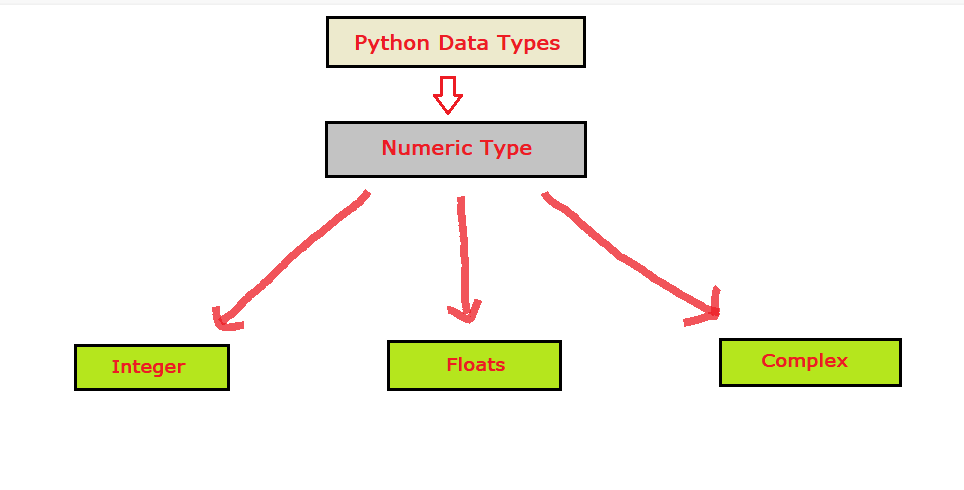# Python Numbers, Type Conversion, and Mathematics

Python is an interpreted, object-oriented, high-level programming language with dynamic semantics. It is an open-source language.

Python Numbers:

There are several numeric data types in python let’s understand them one by one. In python, there is no need to define a data type of any of the variables. Once you assign a value to a particular variable it automatically detects its datatype.

E.g: A variable of numeric type will be created once you assign a value to them.

Types of numbers in Python:

• Int
• Float
• ComplexCode:

## Python Code

``````# integer type
variable1 = 5

# float type
variable2 = 3.142

# complex type
variable3 = 5j

# type() method return the type of variable
# data type of the variable
print(type(variable1))
print(type(variable2))
print(type(variable3))
``````

Output:

<class ‘int’>
<class ‘float’>
<class ‘complex’>

### Type conversion in python:

Sometimes we need to convert one type of data to another type to meet requirements. This is achieved with the help of type conversion.

Type conversion is a technique of converting the value of one data type i.e.(integer, complex, float, etc.) to another data type.

Type conversion in python is divided into two parts:

1. Implicit type conversion:- In Implicit type conversion, the python interpreter itself converts the type of a variable to another type without the user’s consent.
1. Explicit type conversion:- In Explicit type conversion, the user can explicitly change the data type of the variables. Python has the following built-in functions for type conversion:
• int(): To convert any number or string of numbers to an integer.
• float(): To convert numbers to floating-point.
• complex(): To convert two numbers to a complex number.

Code:

## Python Code

``````var1 = 1.2 #float
var1 = int(var1)  #convert 1.2 i.e float to integer
print(type(var1)) #integer

var2 = 1 #integer
var2 = float(var2)  #convert 1 i.e integer to floating point type
print(type(var2)) #float

var3 = 1 #integer
var4 = 2 #integer
var5 = complex(1,2) #convert 1 and 2 i.e integers into complex number
print(type(var5)) #complex
``````

Output:

<class ‘int’>
<class ‘float’>
<class ‘complex’>

### Python Mathematics

Python has a variety of modules like math and random to carry out different mathematical calculations and operations like trigonometry, logarithms, probability, statistics, etc.

The math module in python is a standard module and is always available. To use this module in our program we just have to simply import it using the command import math and it gives access to all the different functions present in the module.

Code:

## Python Code

``````# importing math module in our program
import math

#square root of 4
print("The square root of 4 is:")
print(math.sqrt(4))
print("\n")

#value of pi
print("Value of pi is:")
print(math.pi)
print("\n")

#trigonometric cos value of pi
print("The trigonometric cos value of pi is: ")
print(math.cos(math.pi))
print("\n")

#value of log of 1000 to the base 10
print("Value of log of 1000 to the base 10 is: ")
print(math.log10(1000))
print("\n")

#factorial of 5
print("Factorial of 5 is: ")
print(math.factorial(5))
``````

Output:

The square root of 4 is:
2.0

Value of pi is:
3.141592653589793

The trigonometric cos value of pi is:
-1.0

Value of log of 1000 to the base 10 is:
3.0

Factorial of 5 is:
120

Below is the list of some functions that are available in the math module of python with their description.

Special thanks to Abhishek Yadav for contributing to this article on takeUforward. If you also wish to share your knowledge with the takeUforward fam, please check out this articleIf you want to suggest any improvement/correction in this article please mail us at [email protected]